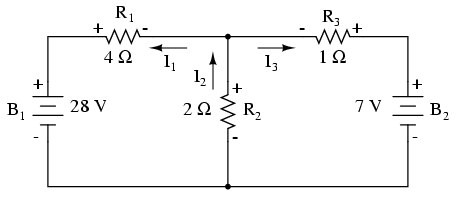My Math ForumHow to calculate current through Resistance
 User Name Remember Me? Password

 Physics Physics Forum

 June 15th, 2016, 10:22 PM #1 Banned Camp   Joined: May 2016 From: earth Posts: 703 Thanks: 56 How to calculate current through Resistance Hello,in this circuit i applied KCL and KVL i get these equation I1 +I3 - I2=0 eq1 KCL 28 - 4*I1 - 2*I2=0 eq 2 KVL 7 - I3 - 2*I2 =0 eq 3 KVL how to get individual value.June 16th, 2016, 12:20 AM #2 Senior Member   Joined: Apr 2014 From: UK Posts: 965 Thanks: 342 Use substitution. The first equation can be rearranged as I2 = I1 + I3, so start with replacing I2 in equations 2 and 3. You will then be able to rearrange the 2nd equation and substitute it in the 3rd.June 16th, 2016, 01:44 AM #3 Banned Camp   Joined: May 2016 From: earth Posts: 703 Thanks: 56 I1 +I3 - I2=0 eq1 KCL 28 - 4*I1 - 2*I2=0 eq 2 KVL 7 - I3 - 2*I2 =0 eq 3 KVL I1 +I3 = I2 28 - 4*I1 - 2*(I1+I3) 28- 4I1 - 2I1 -2I3 28 - 6I1 - 2I3 eq4 7 - I3 - 2*I2 =0 7-I3 - 2(I1+I3) 7-I3-2I1- 2I3 7-3I3 - 2I1 eq 5 28= 6I1 + 2I3 7=3I3 + 2I1 28= 6I1 + 2I3 7=2I1 + 3I3 * 3 28= 6I1 + 2I3 21=6I1 + 9I3 7=-7I3 I3=-1Amp 7 - I3 - 2*I2 =0 7 + 1 - 2*I2 =0 I2 = 4Amp 7 - I3 - 2*I2 =0 7 - I3 - 2*4 =0 7 -I3 -8=0 -1 -I3=0 I3=1amp but in simulation answer is 5,1,4ampsJune 16th, 2016, 03:43 AM #4 Senior Member   Joined: Apr 2014 From: UK Posts: 965 Thanks: 342 Equations 2 and 3 are wrong, some of the currents are drawn the opposite way to 'normal' eq2: 28 - -4I1 - -2I2 = 28 + 4I1 + 2I2 = 0 eq3: 7 - -I3 - -2I2 = 7 + I3 + 2I2 = 0June 16th, 2016, 03:53 AM   #5
Banned Camp

Joined: May 2016
From: earth

Posts: 703
Thanks: 56

Quote:
 Equations 2 and 3 are wrong, some of the currents are drawn the opposite way to 'normal'
i have taken current from the source to load as it do..June 16th, 2016, 05:03 AM #6 Senior Member   Joined: Apr 2014 From: UK Posts: 965 Thanks: 342 The voltages in the left hand loop are: 28V - 0V = 28V Voltage at [R1-] - voltage at [R1+] = --4I1 = 4I1 Voltage at [R2-] - voltage at [R2+] = 0 - - voltage at R2+ = 2I2 Note that the currents in R1 and R2 will be negative as measured in the direction shown. Thanks from MMathJune 16th, 2016, 05:42 AM #7 Banned Camp   Joined: May 2016 From: earth Posts: 703 Thanks: 56 i dont understand the current is from positive terminal to negative but here is difference.June 16th, 2016, 06:21 AM #8 Senior Member   Joined: Apr 2014 From: UK Posts: 965 Thanks: 342 The arrows show the direction of measurement, either way around works, the sign just changes. Maybe try a simpler circuit, take B2 and R3 out of the circuit and apply the rules to that (B1, R1 and R2 only). Thanks from MMathJune 16th, 2016, 06:47 AM   #9
Banned Camp

Joined: May 2016
From: earth

Posts: 703
Thanks: 56

Quote:
 apply the rules to that (B1, R1 and R2 only).
then i can do.
it will behave like superposition theorem.Tags calculate, current, resistanceThread ToolsShow Printable VersionEmail this Page Display ModesLinear ModeSwitch to Hybrid ModeSwitch to Threaded ModeSimilar Threads Thread Thread Starter Forum Replies Last Post dave daverson Algebra 1 October 28th, 2012 06:35 PM arron1990 Physics 5 March 28th, 2012 05:52 AM oswaler Physics 1 March 19th, 2008 07:36 PM dave daverson Calculus 1 December 31st, 1969 04:00 PM

 Contact - Home - Forums - Cryptocurrency Forum - Top